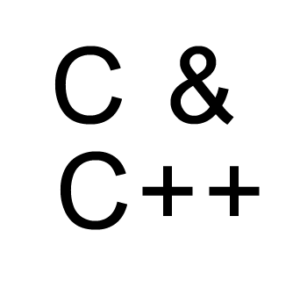## Archives for C and C++ Programming## Addition, subtraction, multiplication and division of two numbers in c programming

Addition, subtraction, multiplication and division of two numbers in c programming In this post we will show you how to Addition, subtraction, multiplication and division of two numbers in c…
Continue Reading
12